# Convert Date and Time from GMT (Greenwich Mean Time) to CST (Central Standard Time)

## Convert GMT to CST

Central Standard Time (CST) is 6 hours behind Greenwich Mean Time (GMT) or Universal Coordinated Time (UTC, sounds familiar no).

So, to convert GMT to CST we just need to subtract 6 hours from GMT time. Let’s do it...

## Generic Formula For (hh:mm:ss AM/PM) Format

 =Timestamp + 1 - TIME(6,0,0)

Example:

I have GMT timestamps in range A2 to A4 and I want CST time in range B2 to B4.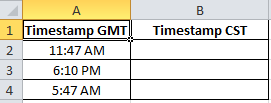We just need to subtract 6 hours from A2 to successfully convert GMT to CST. We will use the TIME() function of excel.

The formula is quite simple. In cell B2, write this formula.

 =A2+1-TIME(6,0,0)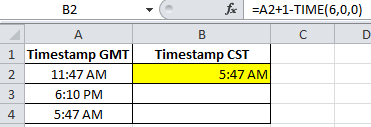Explanation:

The formula is simply subtracting 6 hours, 0 minutes and 0 seconds to convert GMT to CST.

You must be wondering why there is an additional +1. Don’t worry I got you.

Why +1?

This is to avoid any errors. Let’s say you want to convert 5:0 0 AM into CST. You subtract 6 hours and you expect to get to 11:00 PM. But instead of that, you get ######. You don’t want this, right?

When you just enter time in a cell without any date, excel by default takes 1/0/1900 as date, which is excel’s first date. Excel doesn’t know the history before that. Don’t try to teach him. He gets #######. So when you subtract 6 hours from 1/0/1900 5:00 AM it results in an error since there are only 5 hours available.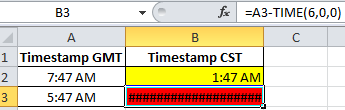+1 adds 24 hours to the given time that prevents error.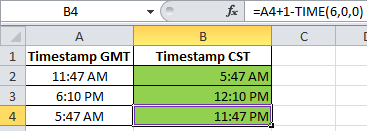## Generic Formula For (MM/DD/YYYY hh:mm:ss AM/PM) Format

 =Timestamp - TIME(6,0,0)

You can simply subtract 6 hours using TIME() function to convert GMT into CST in excel 2016, 2013,and 2010.

Example:

I have GMT time in Range (A2:A4).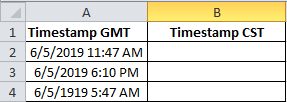To get it converted into CST time zone we just need to enter the formula below into B2 and then drag it down.

 =A2-TIME(6,0,0)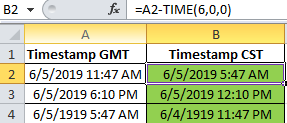You must have noticed that we don’t have that additional +1 in the formula, why?

Right, because we have a date associated with that time.

To know what time is it now central time just use above Formula with NOW() function

 =NOW() - TIME(6,0,0)

You can use this formula to make a live GMT to CST converter.

Convert CST to GMT

To convert CST to GMT time just add 6 hour in CST time. As I explained, Central Time is 6 hour behind Greenwich Mean Time, we just need to add 6 hours to convert CST to GMT.

To convert CST to GST time just replace minus (-) sign with plus (+) sign.

## Generic Formula For (hh:mm:ss AM/PM) Format

 =Timestamp + 1 + TIME(6,0,0)

## Generic Formula For (MM/DD/YYYY hh:mm:ss AM/PM) Format

 =Timestamp - TIME(6,0,0)

I’m sure  that I have cleared your thoughts regarding the GMT to CST and CST to GMT time conversion in excel 2016, 2013 and 2010. If you still have any questions, the comments section is open for you.

Terms and Conditions of use

The applications/code on this site are distributed as is and without warranties or liability. In no event shall the owner of the copyrights, or the authors of the applications/code be liable for any loss of profit, any problems or any damage resulting from the use or evaluation of the applications/code.